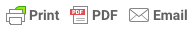# Sampling Distribution

### Sampling Distribution

By , published 2019

Sample statistics only estimate population parameters, such as the mean or standard deviation. This is because in real-world research only a sample of cases are selected from the population, as due to time restraints and practical issues a researcher cannot test the total population.

Therefore, it is likely that the sample mean will be different to the (unknown) population mean. However, a researcher will never know the exact amount of sampling error, but by using a sampling distribution they can estimate the sampling error.

###### What is a sampling distribution?

A sampling distribution is a probability distribution of a statistic (such as the mean) that results from selecting an infinite number of random samples of the same size from a population. The sampling distribution of a given population is the distribution of frequencies of a range of different outcomes that could possibly occur for a statistic of a population.

To create a sampling distribution a research must (1) select a random sample of a specific size (N) from a population, (2) calculate the chosen statistic for this sample (e.g. mean), (3) plot this statistic on a frequency distribution, and (4) repeat these steps an infinite number of times.

It is important to note that sampling distributions are theoretical, and the researcher does not select an infinite number of samples. Instead, they conduct repeated sampling from a larger population., and use the central limit theorem to build the sampling distribution.

Three different distributions are involved in building the sampling distribution.

1. The population distribution from which the random samples are selected.
2. The infinite number of random samples that are selected.
3. The sampling distribution that is being created.

###### Why is the Central Limit Theorem so important to the study of sampling distribution?

The central limit theorem tells us that no matter what the distribution of the population is, the shape of the sampling distribution will approach normality as the sample size (N) increases.Figure 1. Distributions of the sampling mean (Publisher: Saylor Academy).

This is useful, as the research never knows which mean in the sampling distribution is the same as the population mean, but by selecting many random samples from a population the sample means will cluster together, allowing the research to make a very good estimate of the population mean.

Thus, as the sample size (N) increases the sampling error will decrease.